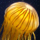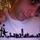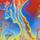## General Question# What's the central limit theorem allow you to do?

Asked by stopman (5) September 25th, 2009

I’m interested in what the counterintuitive result of the central limit theorem says, which is: when you randomly sample from a distribution (even if the distribution is non-normal) the means will be normally distributed. Does that enable you to use other statistical tools? Basically I’m asking what that result means for applications if your distribution is non-normal.

Observing members: 0Composing members: 0I can’t offer much here, since I haven’t done statistics in a while. I was just having a conversation recently with a statistician, and they mentioned that this technique of taking multiple subsamples of a given sampling allowed you to simply get results that would otherwise require much deeper and more difficult statistical techniques. So I think the point is that you can use relatively easy statistical tools even in your sample doesn’t have a normal distribution.

Sorry I can’t give you more details on this.

finkelitis (1907)“Great Answer” (0) Flag as…The central limit theorem allows you to sample a distribution, take averages, and analyze the averages, even if you don’t know the shape of the original distribution. This is because the distribution of the averages is always normal, even if the original distribution is not.

There are a wide variety of practical applications for this theoem. For example, let’s say you work at Campbell’s soup and you want to make sure there is exactly 10oz of soup in a can. Campbell’s produces way more cans than you can sample, and you don’t know the distribution of the contents of the cans; but you can sample five per hour, take the average of the five and plot that value. You can analyze the distribution of the averages, and look for patterns and outliers using statistics for normal distributions, without ever knowing the original distribution.

PerryDolia (3470)“Great Answer” (1) Flag as…or